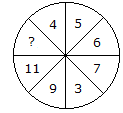# Verbal Reasoning - Character Puzzles - Discussion

### Discussion :: Character Puzzles - Character Puzzles 1 (Q.No.11)

11.

Which one will replace the question mark ?[A]. 13 [B]. 14 [C]. 12 [D]. 15

Explanation:

Sum of numbers in lower half of the circle = 11 + 9 + 3 + 7 = 30

Sum of numbers in upper half of the circle = ? + 4 + 5 + 6 = ? + 15

Upper half = Lower half

30 = ? + 15

? = 30 - 15

? = 15.

 Priyanshu said: (Aug 12, 2016) I don't think logic is correct in this question. Can someone explain it in another way?

 Prasanta said: (Oct 4, 2016) I didn't understand, can anyone explain it in another way?

 Lily said: (May 16, 2017) According to me, The answer is 10.

 Sanju said: (Jun 15, 2017) 3+4 = 7 4+5 =9 5+6 = 11 6+7 = 13 So ans 13.

 Ramsingh said: (Aug 8, 2017) ?+4+5+6 = 11+9+3+7. ?+15 = 30. ? = 30 -15, ? = 15 anwer.

 Monalisha said: (Dec 8, 2020) Please, anyone tell me the correct answer.

 Arun said: (Jul 13, 2021) But how can we assume that they would be equal? Please explain.